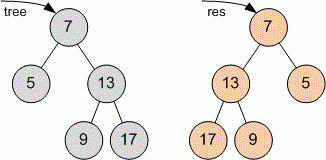Competitions

# Tree Invert

Given a binary tree. Invert it. For each node:

• left child must become right child;
• right child must become left child;

Definition of a tree:

// Java
class TreeNode
{
public:
int val;
TreeNode left;
TreeNode right;
TreeNode(int x) {
val = x;
left = NULL;
right = NULL;
};

// C++
class TreeNode
{
public:
int val;
TreeNode *left;
TreeNode *right;
TreeNode(int x) : val(x), left(NULL), right(NULL) {}
};


Implement function Invert that returns the pointer to the inverted tree.

// Java
TreeNode Invert(TreeNode tree)

// C++
TreeNode* Invert(TreeNode *tree)


#### ExampleFunction Invert returns res - pointer to the inverted tree.

Time limit 1 second
Memory limit 128 MiB
Author Mykhailo Medvediev